## Ultrametric

An ultrametric is a Metric which satisfies the following strengthened version of the Triangle Inequality,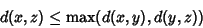for all. At least two of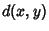,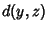, and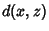are the same.

Letbe a Set, and let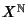(where N is the Set of Natural Numbers) denote the collection of sequences of elements of(i.e., all the possible sequences,,, ...). For sequences,, letbe the number of initial places where the sequences agree, i.e.,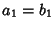,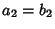, ...,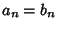, but. Takeif. Then defininggives an ultrametric.

The p-adic Number metric is another example of an ultrametric.